# Grade - math word problems

#### Number of problems found: 5415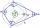What size have angles in quadrilateral (4-gon) if they are in a ratio of 8: 9: 10: 13?
• Equilateral cylinder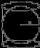A sphere is inserted into the rotating equilateral cylinder (touching the bases and the shell). Prove that the cylinder has both a volume and a surface half larger than an inscribed sphere.
• Blueberries 2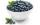Lenka and Martin collected eight liters of blueberries in two hours. How long will take to collect blueberries by Lenka itself, if we know that she collect all 2.5 hours less than Martin?
• Cuboid - volume and areasThe cuboid has a volume of 250 cm3, a surface of 250 cm2 and one side 5 cm long. How do I calculate the remaining sides?
• Bath salts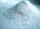Bath salt A weighs 900 grams and costs 36,40 CZK. Bath Salts B weighs 1000 grams, but costs 85 CZK. How much is a bath salt A cheaper than B one?
• Sum or productWhat is the probability that two dice fall will have the sum 7 or product 12?
• BricksOpenings in perforated bricks occupy 10% and brick has dimensions 30 cm, 15 cm and 7.5 cm. Calculate a) the weight of a perforated bricks, if you know that the density of the full brick material is p = 1800 kg/m3 (1.8 kg/dm3) b) the number of perforated
• Wide fieldThe field is 203 meters wide 319 meter long, what is the greater length of the rope by which length and width can be measured and find the exact number of time.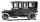Car started at 11:40 at speed 54 km/h from A and to B arrived at 12:20. Determine the distance A and B.
• RectangleCalculate area of the rectangle if its length is 12 cm longer than its width and length is equal to the square of its width.
• Ice cream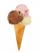Annie likes much ice cream. In the shop are six kinds of ice cream. In how many ways she can buy ice cream to three scoop if each have a different flavor mound and the order of scoops doesn't matter?
• Three dice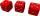What is the probability that when we roll three dice, we roll the numbers 1,2,3?
• NumberI think the number. If I add to its third seven I get same as when to its quarter add 8. Which is the number?
• TilesThe room has dimensions 12 m and 5.6 m. Determine the number of square tiles and their largest possible size to cover them room's floor.
• One and a half chicken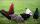One and a half chicken bears one and a half egg in one and a half day. How many eggs can withstand from 6 chicken in 6 days?
• Three numbers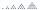What is three numbers which have the property: the sum of the reciprocals of the first and second numbers is 12/7, the first and third 11/24 and the second and the third 3/8.
• Raffle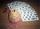How many raffle tickets must be purchased by Peter in raffle with issued 200 tickets if he wants to be sure win at least 3 price? In the raffle draws 30 prices.
• Diagonals in diamons/rhombusRhombus ABCD has side length AB = 4 cm and a length of one diagonal of 6.4 cm. Calculate the length of the other diagonal.
• Company accountThe company had an account of EUR 10,000. On Monday it withdraw 3,000 euros and Tuesday 6,000 euros. How much did the euro remain in the account?
• Three digits numberFrom the numbers 1, 2, 3, 4, 5 create three-digit numbers that digits not repeat and number is divisible by 2. How many numbers are there?

Do you have an interesting mathematical word problem that you can't solve it? Submit a math problem, and we can try to solve it.

We will send a solution to your e-mail address. Solved examples are also published here. Please enter the e-mail correctly and check whether you don't have a full mailbox.

Please do not submit problems from current active competitions such as Mathematical Olympiad, correspondence seminars etc...